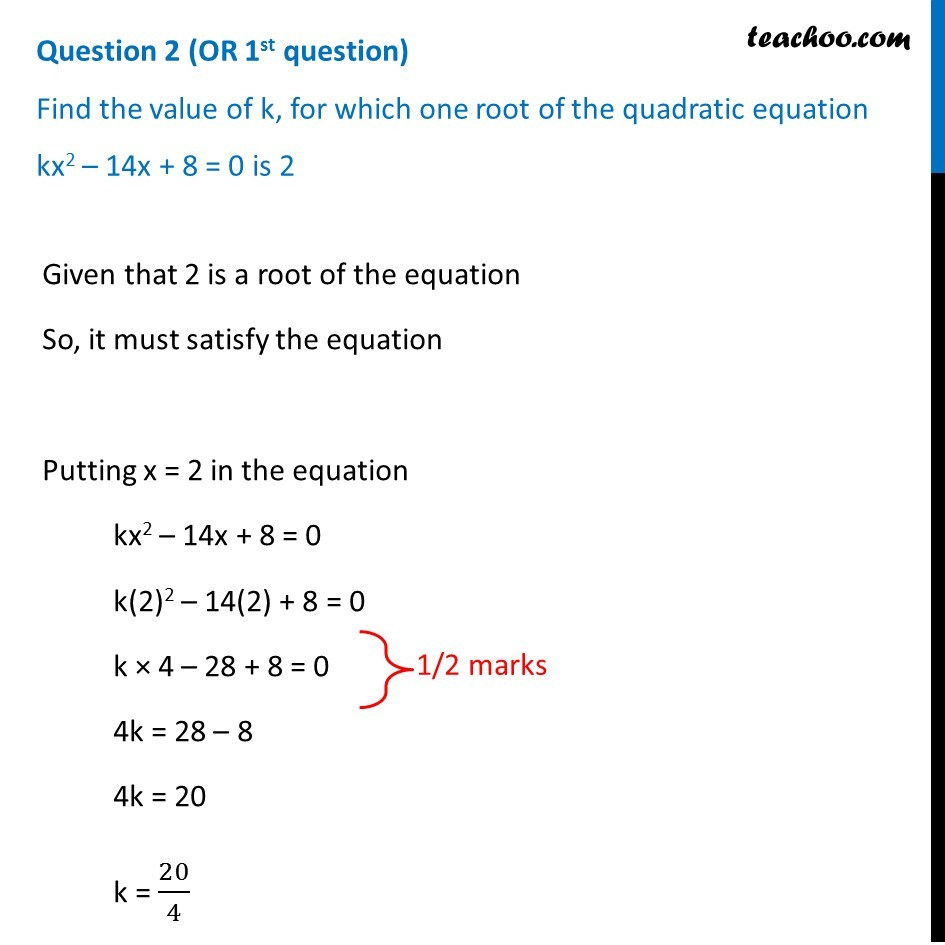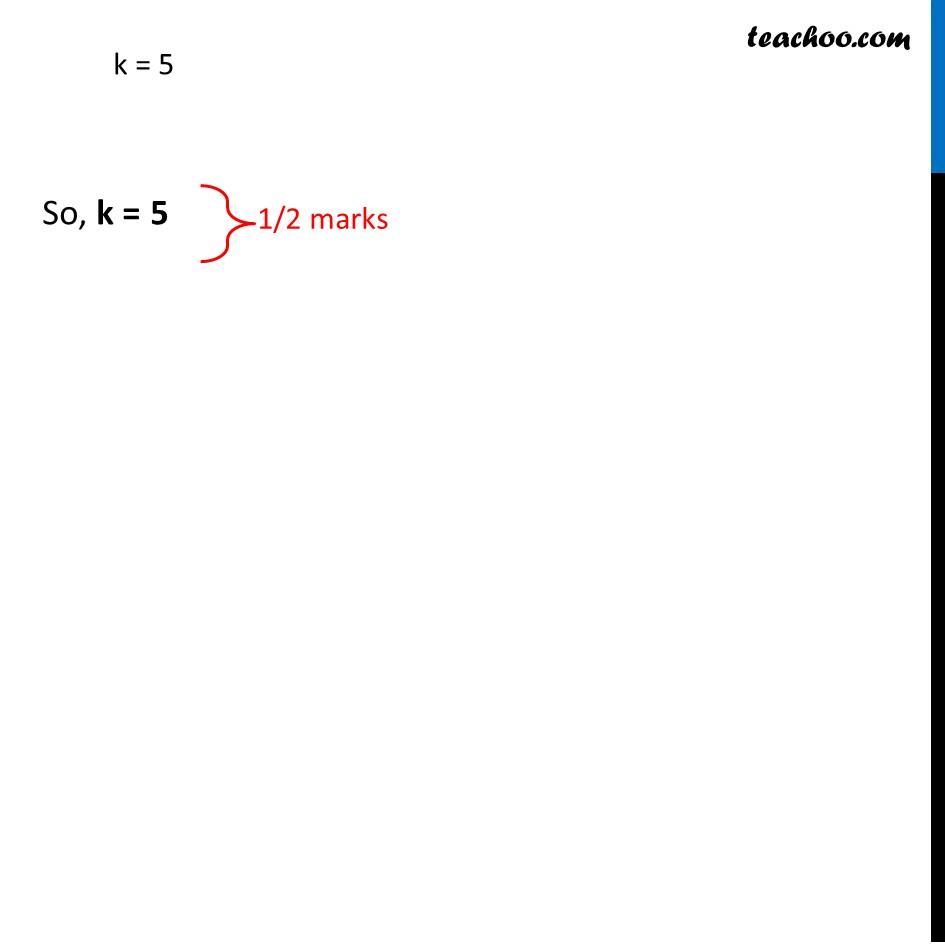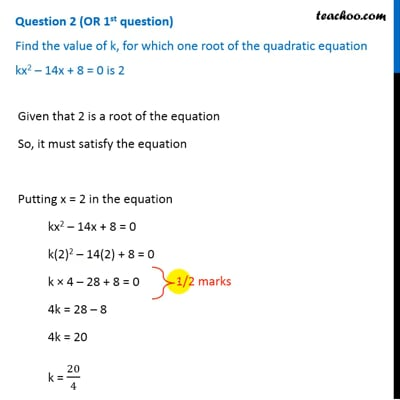CBSE Class 10 Sample Paper for 2019 Boards

Class 10
Solutions of Sample Papers for Class 10 Boards

Question 2 (OR 1 st question)

Find the value of k, for which one root of the quadratic equation kx 2 – 14x + 8 = 0 is 2This video is only available for Teachoo black users

Get live Maths 1-on-1 Classs - Class 6 to 12

### Transcript

Question 2 (OR 1st question) Find the value of k, for which one root of the quadratic equation kx2 – 14x + 8 = 0 is 2 Given that 2 is a root of the equation So, it must satisfy the equation Putting x = 2 in the equation kx2 – 14x + 8 = 0 k(2)2 – 14(2) + 8 = 0 k × 4 – 28 + 8 = 0 4k = 28 – 8 4k = 20 k = 20/4 k = 5 So, k = 5